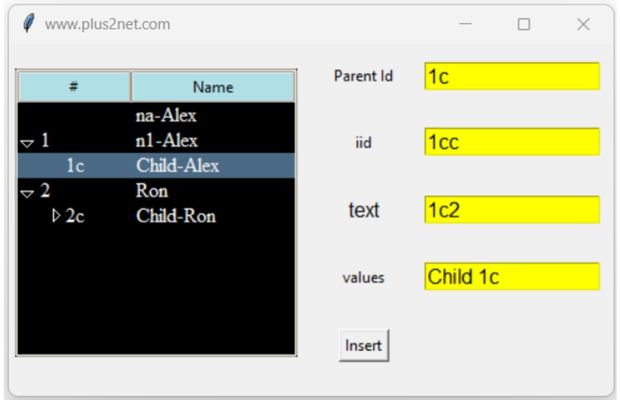# Parent Child in Tkinter Treeview

Treeview
Tkitner Treeview to add parent and child rows using insert() with parent and iid options

We will extend the same code taken from our basic Treeview tutorial.To this code we will add few more rows where some rows are child of parent row. The identification of parent and child is done by the values for parent option and the iid.
The child will have to iid of parent as option.

## open=True/False

By default the child row can be displayed by using open=True. If this value is set to False then child will be in collapsed state, however it can be displayed by expanding the Tree ( + symbol ) in tree view and by double clicking in headers view.

## show option

`headings`: Show the headings without the column marked as #0
`tree`: Show tree view with expanding the child node. No header row.
`tree headings` : (Default) Show both heading and expanding child node.
`''` : Neither tree view nor headings are shown.
The full code with parent - child in Treeview is here.
``````from tkinter import ttk
import tkinter as tk
# Creating tkinter my_w
my_w = tk.Tk()
my_w.geometry("400x280")
my_w.title("www.plus2net.com")
# Using treeview widget
trv = ttk.Treeview(my_w, selectmode ='browse')

# number of columns
trv["columns"] = ("1", "2")

#trv['show'] = 'tree'
s=ttk.Style()
s.configure(trv,rowhieght=20)
s.configure(trv,height=20)
# width of columns and alignment
trv.column("#0", width = 100, anchor ='c')
trv.column("1", width = 60, anchor ='c')
trv.column("2", width = 100, anchor ='c')

# respective columns

trv.insert("", 'end',iid='a',open=True,
values =('a','na-Alex'))
trv.insert("", 'end',iid=1,open=True,text='1',
values =(1,'n1-Alex'))
trv.insert("1", 'end',iid='1c',open=True,text='1c',
values =('1c','Child-Alex'))
trv.insert("", 'end',iid=2, open=True,text=2,
values =(2,'Ron'))
trv.insert("2", 'end',iid='2c',open=False,text='2c',
values =('2c','Child-Ron'))
trv.insert("2c", 'end',iid='2cc', open=True,text='2cc',
values=('2cc','Child-2-Ron'))

my_w.mainloop()``````

## Getting list of data from Treeview

``````my_list=[]
for child in trv.get_children():
my_list.append(trv.item(child)["values"])
#print(trv.item(child)["values"])
#print(trv.item(child)["values"]) # for Name
print(my_list)``````

## Inserting User entered dataBy using Entry widgets we can add row data to Treeview. Here based on the selection of the row, the input data will be stored as child row or can be stored as root or parent row.
On selection of any row of the Treeview, we can collect the iid ( parent id ) of the row and kept as default value for Parent id input. User can change the parent id or keep as blank to set the input data as root or parent row.

Inserting user input data as child or parent item to a Tkinter Treeview by using Entry widgets

``````from tkinter import ttk
import tkinter as tk
# Creating tkinter my_w
my_w = tk.Tk()
my_w.geometry("480x280")
my_w.title("www.plus2net.com")
# Using treeview widget
font1=['Times',12,'normal']
style = ttk.Style(my_w)
style.theme_use("clam") # set theam to clam
style.configure("Treeview", background="black",
fieldbackground="black", foreground="white",font=font1)

trv = ttk.Treeview(my_w, selectmode ='browse')
# number of columns
trv["columns"] = ("1")
#trv['show'] = 'tree'

# width of columns and alignment
trv.column("#0", width = 90, anchor ='w')
trv.column("1", width = 130, anchor ='w')

# respective columns
trv.insert("",'end',iid='a',open=True,values=('na-Alex'))
trv.insert("",'end',iid=1,open=True,text='1',values=('n1-Alex'))
trv.insert("1",'end',iid='1c',open=True,text='1c',values =('Child-Alex'))
trv.insert("", 'end',iid=2, open=True,text=2,values =('Ron'))
trv.insert("2",'end',iid='2c',open=False,text='2c',values =('Child-Ron'))
trv.insert("2c",'end',iid='2cc',open=True,text='2cc',values=('Child2-Ron'))

def data_collect(self):
p_id = trv.selection() # collect selected row id
e1_str.set(p_id)

def data_insert():
print(e4_str.get())
if(e2_str.get()==''):
trv.insert(e1_str.get(),'end',open=True,
text=e3_str.get(),values=(e4_str.get(),))
else:
trv.insert(e1_str.get(),'end',iid=e2_str.get(),open=True,
text=e3_str.get(),values=(e4_str.get(),))
e1_str.set('')
e2_str.set('')
e3_str.set('')
e4_str.set('')

trv.bind("<<TreeviewSelect>>", data_collect) # on select event
l1=tk.Label(my_w,text='Parent Id',width=10)
l1.grid(row=1,column=2)
e1_str=tk.StringVar(my_w)
e1=tk.Entry(my_w,textvariable=e1_str,bg='yellow',width=15,font=16)
e1.grid(row=1,column=3)

l2=tk.Label(my_w,text='iid',width=10)
l2.grid(row=2,column=2)
e2_str=tk.StringVar(my_w)
e2=tk.Entry(my_w,textvariable=e2_str,bg='yellow',width=15,font=16)
e2.grid(row=2,column=3)

l3=tk.Label(my_w,text='text',width=10,font=16)
l3.grid(row=3,column=2)
e3_str=tk.StringVar(my_w)
e3=tk.Entry(my_w,textvariable=e3_str,bg='yellow',width=15,font=16)
e3.grid(row=3,column=3)

l4=tk.Label(my_w,text='values',width=10)
l4.grid(row=4,column=2)
e4_str=tk.StringVar(my_w)
e4=tk.Entry(my_w,textvariable=e4_str,bg='yellow',width=15,font=16)
e4.grid(row=4,column=3)
b1=tk.Button(my_w,text='Insert',command=lambda:data_insert())
b1.grid(row=5,column=2)

my_w.mainloop()``````

Subscribe to our YouTube Channel here

## Subscribe

* indicates required
Subscribe to plus2netplus2net.com